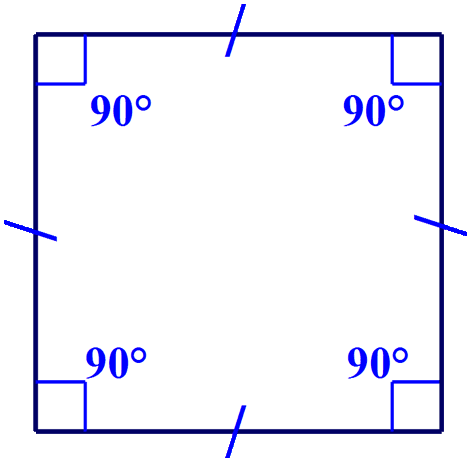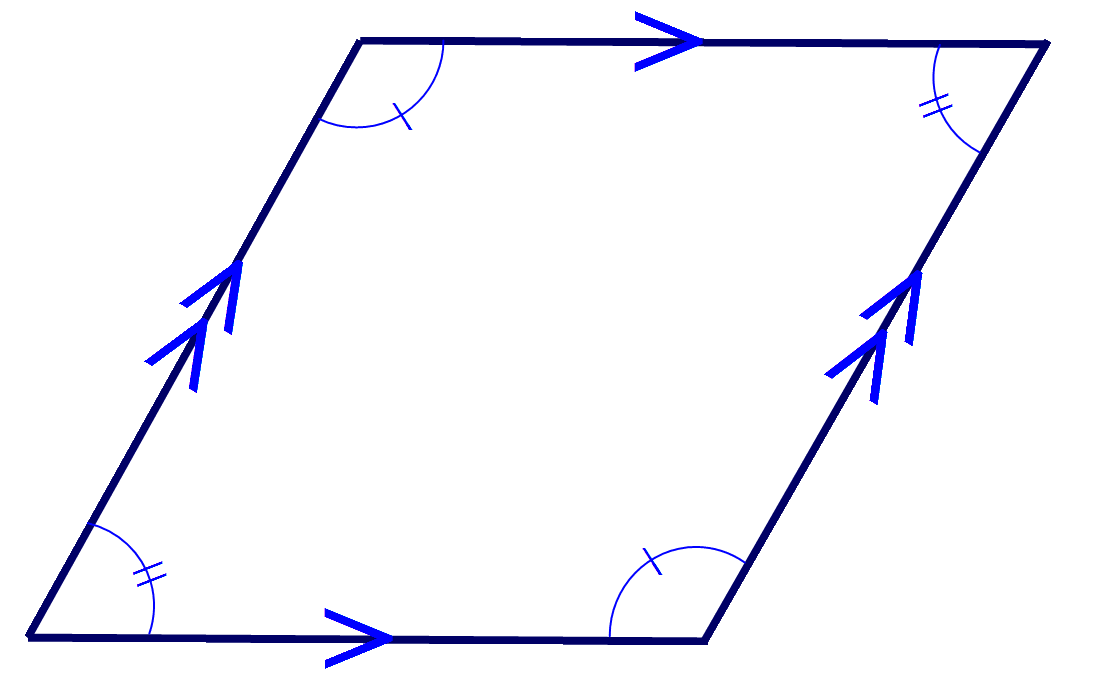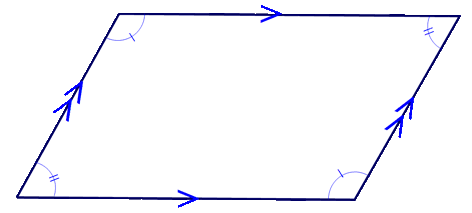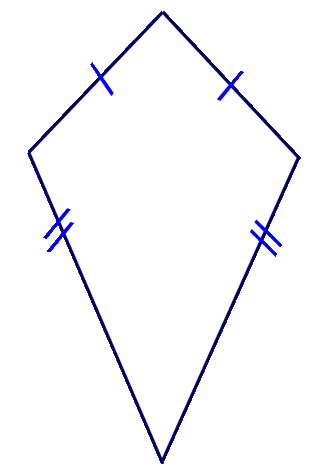### Quadrilaterals are 2 dimensional shapes which have four sides, four angles and four vertices. The four angles of a quadrilateral always add up to 360°.

_________________________________________________________

# Square

## 4 equal angles

The angles of a quadrilateral always add up to 360°, therefore each angle of a square is,
360 ÷ 4 = 90°# Rectangle

## 4 equal angles

The angles of a quadrilateral always add up to 360°, therefore each angle of a rectangle is,
360 ÷ 4 = 90°# Rhombus

## Opposite angles are equal

(like a pushed over square)# Parallelogram

## Opposite angles are equal

(like a pushed over rectangle)# Kite

##_________________________________________________________